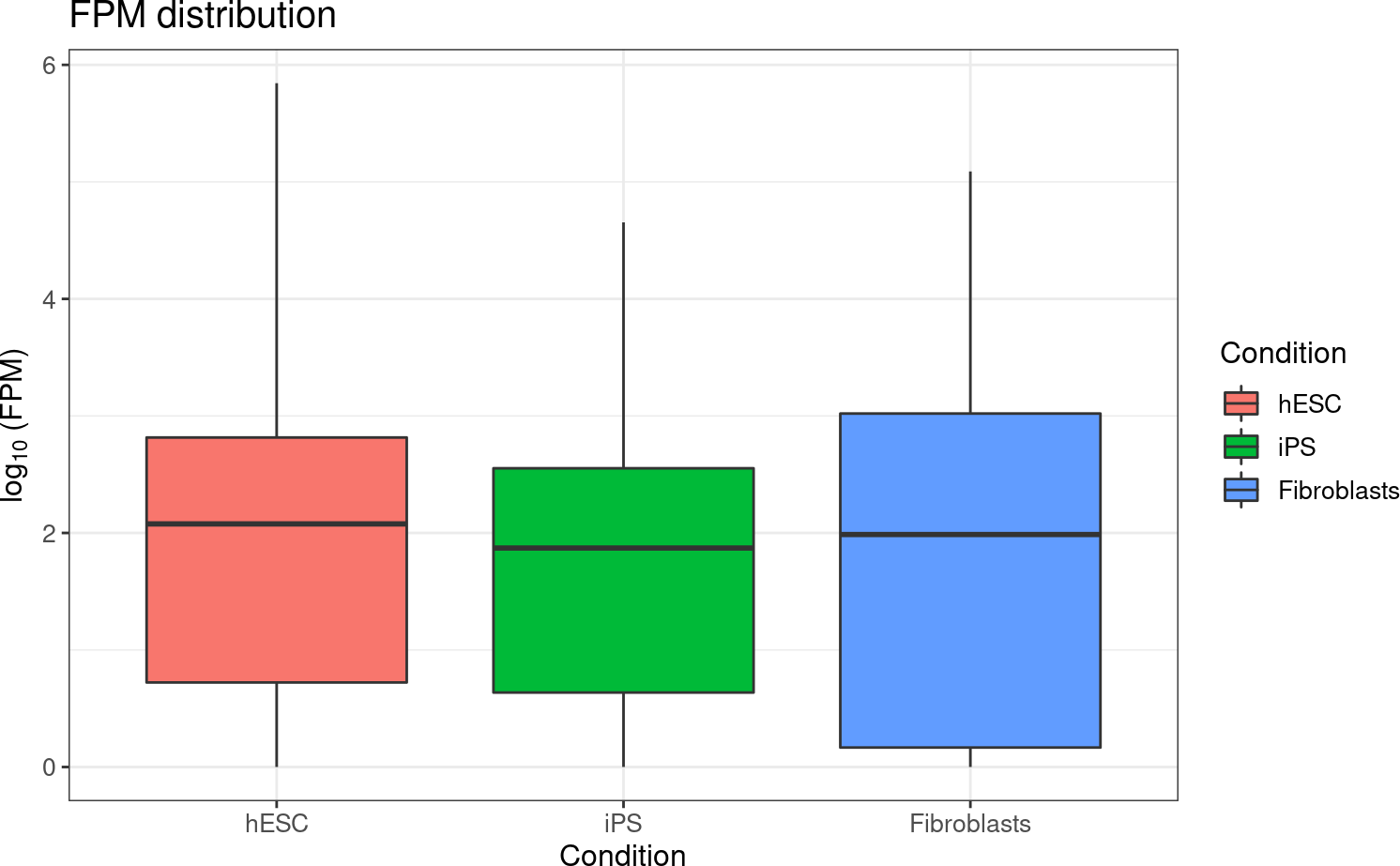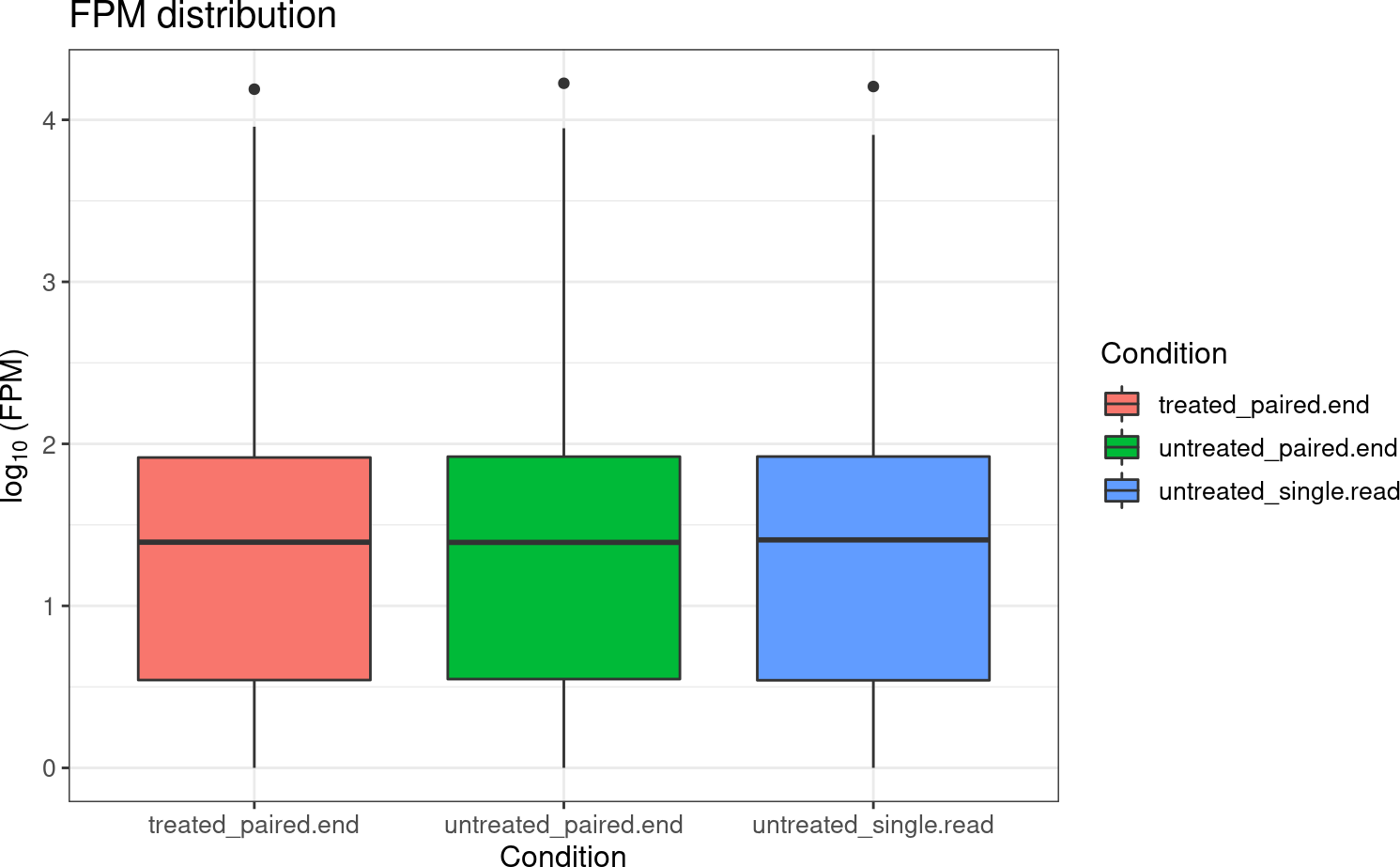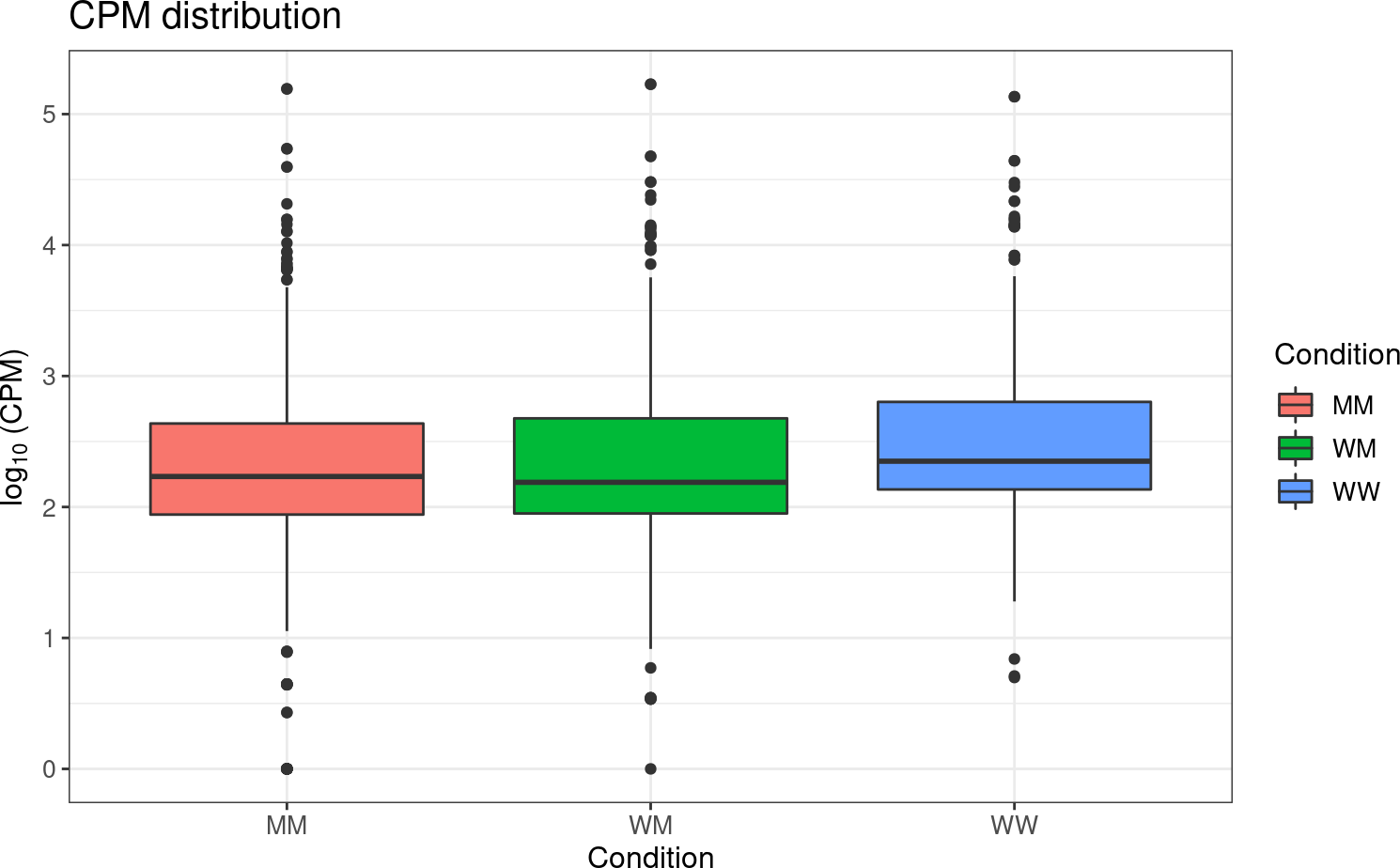# Example S1: Installation and data examples

The stable version of this package is available on Bioconductor. You can install it by running the following:

``````if (!requireNamespace("BiocManager", quietly=TRUE))
install.packages("BiocManager")
BiocManager::install("vidger")``````

The latest developmental version of `ViDGER` can be installed via GitHub using the `devtools` package:

``````if (!require("devtools")) install.packages("devtools")
devtools::install_github("btmonier/vidger", ref = "devel")``````

• `vsBoxplot()`
• `vsScatterPlot()`
• `vsScatterMatrix()`
• `vsDEGMatrix()`
• `vsMAPlot()`
• `vsMAMatrix()`
• `vsVolcano()`
• `vsVolcanoMatrix()`
• `vsFourWay()`

Further explanation will be given to how these functions work later on in the documentation. For the following examples, three toy data sets will be used: `df.cuff`, `df.deseq`, and `df.edger`. Each of these data sets reflect the three RNA-seq analyses this package covers. These can be loaded in the R workspace by using the following command:

``data(<data_set>)``

Where `<data_set>` is one of the previously mentioned data sets. Some of the recurring elements that are found in each of these functions are the `type` and `d.factor` arguments. The `type` argument tells the function how to process the data for each analytical type (i.e. `"cuffdiff"`, `"deseq"`, or `"edger"`). The `d.factor` argument is used specifically for `DESeq2` objects which we will discuss in the DESeq2 section. All other arguments are discussed in further detail by looking at the respective help file for each functions (i.e. `?vsScatterPlot`).

## An overview of the data used

As mentioned earlier, three toy data sets are included with this package. In addition to these data sets, 5 “real-world” data sets were also used. All real-world data used is currently unpublished from ongoing collaborations. Summaries of this data can be found in the following tables:

Table 1: An overview of the toy data sets included in this package. In this table, each data set is summarized in terms of what analytical software was used, organism ID, experimental layout (replicates and treatments), number of transcripts (IDs), and size of the data object in terms of megabytes (MB).

Data Software Organism Reps Treat. IDs Size (MB)
`df.cuff` CuffDiff H 2 3 1200 0.2
sapiens
`df.deseq` DESeq2 D. 2 3 29391 2.3
melanogaster
`df.deseq` edgeR A. 2 3 724 0.1
thaliana

Table 2: “Real-world” (RW) data set statistics. To test the reliability of our package, real data was used from human collections and several plant samples. Each data set is summarized in terms of organism ID, number of experimental samples (n), experimental conditions, and number of transcripts ( IDs).

Data Organism n Exp. Conditions IDs
RW-1 H. 10 Two treatment dosages taken at two 198002
sapiens time points and one control sample
taken at one time point
RW-2 M. 24 Two phenotypes taken at four time 63517
domestia points (three replicates each)
RW-3 V. 6 Two conditions (three replicates 59262
ripria: each).
bud
RW-4 V. 6 Two conditions (three replicates 17962
ripria: each).
shoot-tip
(7 days)
RW-5 V. 6 Two conditions (three replicates 19064
ripria: each).
shoot-tip
(21 days)

# Example S2: Creating box plots

Box plots are a useful way to determine the distribution of data. In this case we can determine the distribution of FPKM or CPM values by using the `vsBoxPlot()` function. This function allows you to extract necessary results-based data from analytical objects to create a box plot comparing \(log_{10}\) (FPKM or CPM) distributions for experimental treatments.

## With Cuffdiff

``````vsBoxPlot(
data = df.cuff, d.factor = NULL, type = 'cuffdiff', title = TRUE,
legend = TRUE, grid = TRUE
)``````Figure 1: A box plot example using the `vsBoxPlot()` function with
`cuffdiff` data. In this example, FPKM distributions for each treatment within an experiment are shown in the form of a box and whisker plot.

## With DESeq2

``````vsBoxPlot(
data = df.deseq, d.factor = 'condition', type = 'deseq',
title = TRUE, legend = TRUE, grid = TRUE
)``````Figure 2: A box plot example using the `vsBoxPlot()` function with
`DESeq2` data. In this example, FPKM distributions for each treatment within an experiment are shown in the form of a box and whisker plot.

## With edgeR

``````vsBoxPlot(
data = df.edger, d.factor = NULL, type = 'edger',
title = TRUE, legend = TRUE, grid = TRUE
)``````Figure 3: A box plot example using the `vsBoxPlot()` function with `edgeR`
data. In this example, CPM distributions for each treatment within an experiment are shown in the form of a box and whisker plot

## Aesthetic variants to box plots

`vsBoxPlot()` can allow for different iterations to showcase data distribution. These changes can be implemented using the `aes` parameter. Currently, there are 6 different variants:

• `box`: standard box plot
• `violin`: violin plot
• `boxdot`: box plot with dot plot overlay
• `viodot`: violin plot with dot plot overlay
• `viosumm`: violin plot with summary stats overlay
• `notch`: box plot with notch

### `box` variant

``````data("df.edger")
vsBoxPlot(
data = df.edger, d.factor = NULL, type = "edger", title = TRUE,
legend = TRUE, grid = TRUE, aes = "box"
)``````